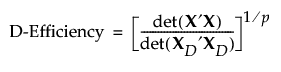Publication date: 11/10/2021

## D-Efficiency

Let X denote the design, or model, matrix for a given assumed model with p parameters. For the definition of the model matrix, see Simulate Responses. Let XD denote the model matrix for a D-optimal design for the assumed model. Then the D-efficiency of the design given by X is as follows: